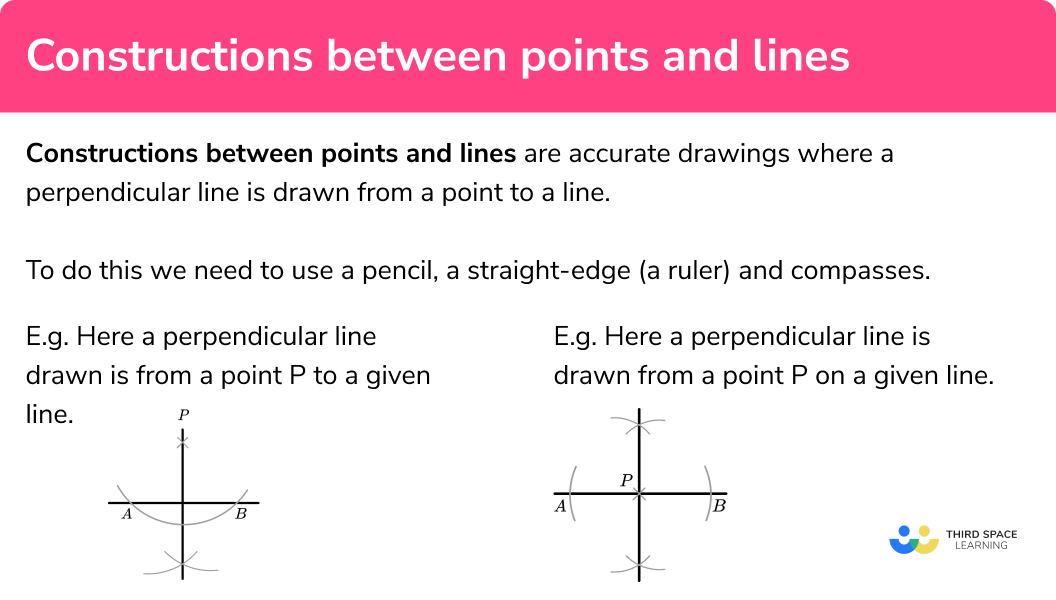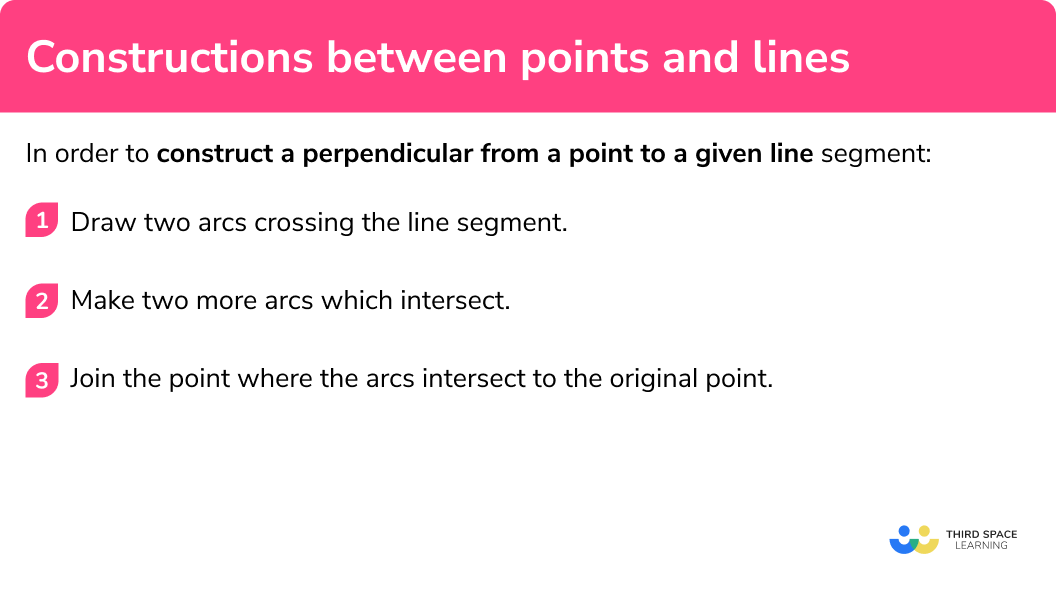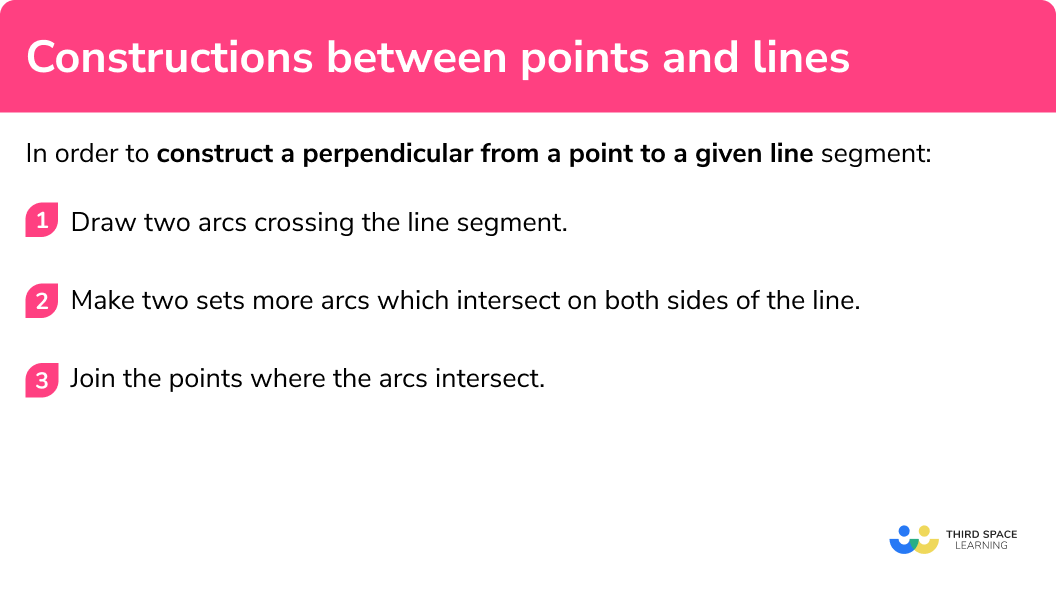GCSE Maths Geometry and Measure Loci And Construction Constructions

Constructions Between Points And Lines

# Constructions Between Points And Lines

Here we will learn about constructions between points and lines, including how to construct a perpendicular from a point to a line using a pencil, a ruler and a pair of compasses.

There are also constructions worksheets, which include constructions between points and lines based on Edexcel, AQA and OCR exam questions, along with further guidance on where to go next if you’re still stuck.

## What are constructions between points and lines?

Constructions between points and lines are accurate drawings where a perpendicular line is drawn from a point to a line.

To do this we need to use a pencil, a straight-edge (a ruler) and compasses.

E.g.

Here a perpendicular line drawn is from a point P to a given line.

Here a perpendicular line is drawn from a point P on a given line.

### What are constructions between points and lines?## How to construct a perpendicular from a point to a line

In order to construct a perpendicular from a point to a given line segment:

1. Draw two arcs crossing the line segment.
2. Make two more arcs which intersect.
3. Join the point where the arcs intersect to the original point.

### How to construct a perpendicular from a point to a line### Related lessons on constructions

Construction between points and lines is part of our series of lessons to support revision on construction and loci and construction. You may find it helpful to start with the main loci and construction lesson for a summary of what to expect, or use the step by step guides below for further detail on individual topics. Other lessons in this series include:

## Constructions between lines and points examples

### Example 1: from a point to a line

Construct a line from point P perpendicular to the line

1. Draw two arcs crossing the line segment.

Put the point of the compasses on the original point P .

Draw an arc that crosses the original line in two places. These are labelled A and B .

2Make two more arcs which intersect.

Put the point of the compasses on point A where an arc crosses the line and draw another arc. Keep the compasses on the same setting. Repeat with point B , drawing another arc to intersect the arc just drawn.

3Join the point where the arcs intersect to the original point.

Using a straight-edge (a ruler), join up the point where the arcs intersect each other and the original point P .

The new line is perpendicular to the original line segment.

The new line will have also bisected the length AB .

### Example 2: from a point to a line

Construct a line from point P perpendicular to the line

Draw two arcs crossing the line segment.

Make two more arcs which intersect.

Join the point where the arcs intersect to the original point.

## How to construct a perpendicular from a point on a line

In order to construct a perpendicular from a point on a given line segment:

1. Draw two arcs crossing the line segment.
2. Make two sets more arcs which intersect on both sides of the line.
3. Join the points where the arcs intersect.

### How to construct a perpendicular from a point to a line## Constructing a perpendicular from a point on a line examples

### Example 3: from a point on a line

Construct a perpendicular line passing through point P

Draw two arcs crossing the line segment.

Make two sets more arcs which intersect on both sides of the line.

Join the points where the arcs intersect.

### Example 4: from a point on a line

Construct a perpendicular line passing through point P

Draw two arcs crossing the line segment.

Make two sets more arcs which intersect on both sides of the line.

Join the points where the arcs intersect.

### Common misconceptions

• The construction arcs must not be removed

The arcs drawn should be drawn lightly so can be adjusted if needed but they must be visible in your final answer. This is to show that you have used the correct method to draw the perpendicular bisector accurately.

• The pencil should be sharp

A sharp pencil helps your diagram to be accurate.  Using a small pencil in compasses can also be helpful.

### Practice constructions between points and lines questions

1. Construct a perpendicular from point P to the line:The construction arcs should be made with compasses and be visible. The final line should go through both sides of the line and be drawn with a straight-edge.

2. Construct a perpendicular from point P to the line:The construction arcs should be made with compasses and be visible. The final line should go through both sides of the line, going through the original point and the intersection of the arcs.

3. Construct a perpendicular from point P on the line:The construction arcs should be made with compasses and be visible. The final line should go through the original point and be drawn with a straight-edge.

4. Construct a perpendicular from point P on the line:The construction arcs should be made with compasses and be visible. The compasses need to be widened for the second set of arcs. The final line should go through the original point and be drawn with a straight-edge.

### Constructions between points and lines GCSE questions

1. Use a ruler and compasses to construct the perpendicular from point R to the line PQ
You must show your construction lines.(2 marks)For the first arc(s) crossing the line centered on R

(1)For the perpendicular with all construction arcs

(1)

2. Use a ruler and compasses to construct the perpendicular from point P on the line MN
You must show your construction lines.(2 marks)For the first arcs crossing the line MN centered on P

(1)

For the perpendicular with all construction arcs

(1)

## Learning checklist

You have now learned how to:

• Construct a perpendicular line from a point to a given line segment
• Construct a perpendicular line from a point on a given line segment

## Still stuck?

Prepare your KS4 students for maths GCSEs success with Third Space Learning. Weekly online one to one GCSE maths revision lessons delivered by expert maths tutors.

Find out more about our GCSE maths tuition programme.